# Two Sinusoidal Models

#### (A new question of the week)

Two recent questions involved using trigonometric functions to model real-life (or nearly so) situations, one about breathing, the other about a Ferris wheel. Both can be done by writing a sinusoidal function; the second can be done in other interesting ways as well.

## Breathing

The first question is from Terry, at the very end of September:

Please assist with the following question.

In a normal respiratory cycle the volume of air that moves into and out of the lungs is about 500 mL. The reserve and residue volumes of air that remain in the lungs occupy about 2000 mL and a single respiratory cycle for an average human takes about 4 seconds. Find a model for the total volume of air V(t) in the lungs as a function of time.

A little background knowledge of physiological terms would help here, but otherwise it is a typical problem of writing an equation of a sinusoidal function … assuming that is what they want, which may be implied by context.

(Technically, we have no reason to think the function will be exactly sinusoidal, but it seems like a reasonable approximation. In checking the wording of the problem, I found that it is from Stewart’s Single Variable Calculus; the context is not specifically about trigonometric functions, but it follows other problems asking for sinusoidal models.)

Doctor Rick started with our standard request for information, and some suggestions:

Hi, Terry.

As you will have seen on our submission page, we ask you to “Show whatever work you have done, and tell us specifically where you need help.” Because you have not done that, I do not know how best to help you.

The first thing we need to know is what you have been learning; this could say a lot about what type of model you are expected to find. My first thought is to use a sinusoidal function to model the volume of air in the lungs as a function of time. Does this fit with what you’ve been doing?

If it does, then you will need to determine an appropriate periodamplitude, and mean level (vertical offset or shift). The phase of the function (or horizontal shift) is not important here; you can do whatever is easiest.

If you want more help, be sure to show what you have tried, and ask any specific questions you have. Also please tell us what methods you have learned that might be relevant. Assuming this is for a class, what is that class and what topics were most recently covered? (Am I right about trigonometric functions?)

Terry responded with his work:

Hi Rick, thank you for your fast reply. Apologies for not specifying, I am new to the website and I will remember that for next time.

So far I have calculated the period, amplitude, and y-intercept and got the following equation:

V(t) = 2000 + 250 sin(π/2×t)

I believe where I am going wrong is with the mean level, however, I’m unsure on how to calculate for that.

The period, which we’ll call T, is the length of one cycle, given as 4 seconds; this implies that the coefficient of t is $$\frac{2\pi}{T} = \frac{2\pi}{4} = \frac{\pi}{2}$$. Terry has this correct.

The amplitude is half the difference between the highest and lowest values (that is, the difference between either of these and their average); we are told that the difference is 500 mL, so the amplitude is half that, 250. This is the multiplier of the sine function, and it too is correct.

The y-intercept for a sine function is the midline of the function (it would be different for a cosine); that appears to be wrong, and will be our main focus.

Doctor Rick commented on these things:

Thanks. Your period looks correct, as does your amplitude. It’s not entirely clear what some phrases mean, but I think you’re correct that 500 mL moving “into and out of the lungs” means that 500 mL of air is exhaled and then 500 mL of air is inhaled. Thus the difference between minimum and maximum volume is 500 mL and the amplitude is 250 mL.

As for the mean level, I believe that the “reserve and residue volumes” (are these two different things??) refers to the minimum volume — the volume of air that is left in the lungs at the end of an exhalation. From that you can find the maximum, and thus the mean, which will be your offset.

From your work it appears that you took the “reserve and residue volumes” to be the mean volume.

### Getting the vertical shift right

Yes, I believe the “reserve and residue” refers to the minimum volume of air (2000 mL)

From what I gathered, are you suggesting that the y-intercept (mean level) be 2250? So I would have an equation such as:

V(t) = 2250 + 250 sin(π/2×t)

I calculated the mean level with the formula -> [2500 – (2500 – 2000) ÷ 2]

Another question I’d like to ask is, what information given in similar questions indicate that I would use the mean level as the vertical shift rather than (for example) the minimum – in this case 2000 mL. Since I have done similar questions in the past but they never required me to account for the mean level such as this one.

Doctor Rick agreed, and showed a check:

You write:

From what I gathered, are you suggesting that the y-intercept (mean level) be 2250? So I would have an equation such as:

V(t) = 2250 + 250 sin(π/2×t)

I calculated the mean level with the formula -> [2500 – (2500 – 2000) ÷ 2]

That is the sinusoidal function I had in mind. The minimum of V(t) is 2250 + 250(–1) = 2000 mL, while the maximum is 2250 + 250(1) = 2500 mL, 500 mL above the minimum. Both are as required.

The mean value of a simple sinusoid is the mean of the minimum and maximum values (peak and trough). For the sine function, the y-intercept is at the mean value; of course, if you had chosen a cosine function, this would not be the case.

In order to make what we say apply to both sine and cosine formulas, we commonly avoid mention of the y-intercept, and talk instead about the “midline” or “average (mean) value”.

Here is the graph, showing how it is shifted: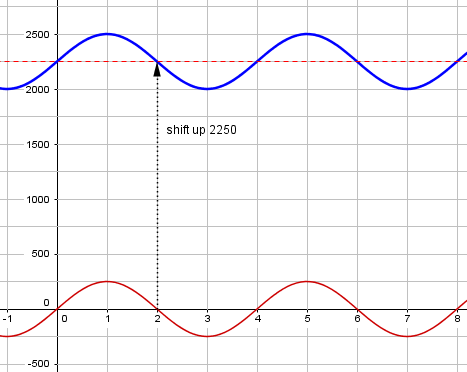Then you write,

Another question I’d like to ask is, what information given in similar questions indicate that I would use the mean level as the vertical shift rather than (for example) the minimum — in this case 2000 mL. Since I have done similar questions in the past but they never required me to account for the mean level such as this one.

I would like to see one of those similar problems in which you didn’t need to account for the mean level, but you did need a vertical shift. You may not have called it the mean level, but you probably used it without thinking of it in these terms.

Perhaps you would refer to the mean value as the “midline” or something like that. It is the horizontal line about which the sinusoid oscillates, equally far above and below it. On an unshifted sinusoid, f(x) = sin(x) or f(x) = cos(x), it is the x-axis. A vertical shift of k transforms the x-axis, y = 0, to the line y = k. That’s the mean value of the transformed sinusoid.

You mention using the minimum value as the vertical shift. That will not work. When we transform y = sin(x) to y = sin(x) + 2, for instance, the minimum value of the transformed function will be -1 + 2 = 1, which is not equal to the vertical offset 2.

In general, a vertical shift moves a given point on the unshifted graph through some fixed vertical distance. For sinusoids, we typically use the midline because that is at $$y=0$$ before shifting. In other cases, such as shifting $$y=x^2$$, we use the minimum (vertex) for the same reason.

Terry replied:

Ah, I see what you mean. You made me realize a critical point that the vertical shift is the mean level in a sinusoidal function. I believe I may have gotten it confused with the cosine graph that starts at the maximum in the mother graph cos(x).

I have done a similar question where the vertical shift was 12 but I also did not realize that 12 was the mean level as well. Thank you for making me notice this important fact that I seemed to have forgotten haha.

I have another question concerning mathematical models and composition of functions – for example f(g(x)). Could I ask it here or should I make an entirely new question?

Doctor Rick closed:

It would be best to submit your question about composition of functions as a new “ticket”. It doesn’t sound like it will depend critically on what we have been discussing.

There is a close connection between transformations of functions (trigonometric or otherwise) and composition of functions; we could have discussed your current problem in terms of composition. I suggest that you read through the following blogs on our site:

Shifting and Stretching Graphs

Finding Transformations from a Graph

Graphing Transformed Sines

When I found the problem in Stewart, it was in a section on function transformations and composition. Now we know Terry’s context!

Terry closed:

Will do so. Thank you so much for your help, Rick!

## A Ferris wheel

The next question came from Kaushal, in mid-October: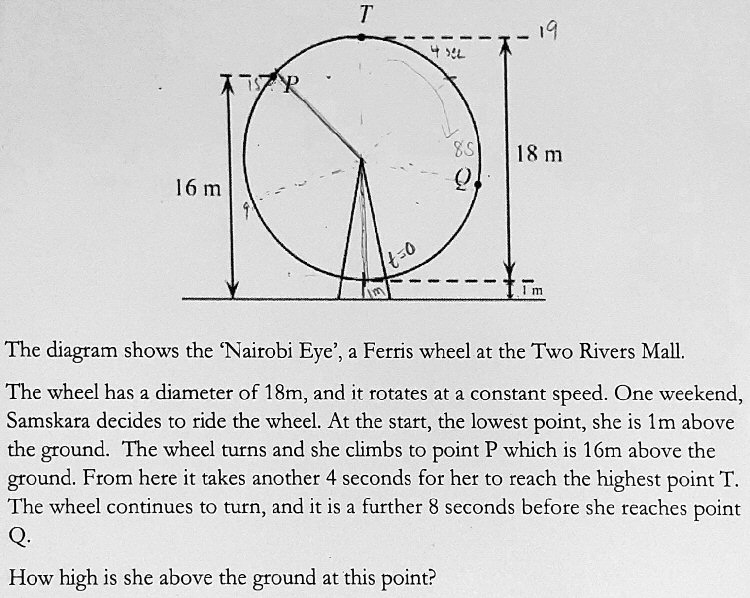Here is my work: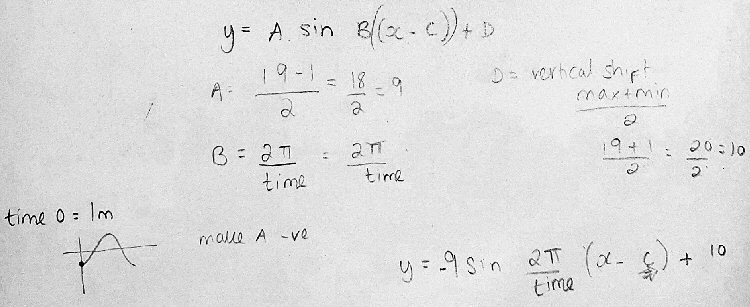Kaushal is doing the same sort of thing Terry did, writing an equation for the sinusoidal motion by determining the required parameters; this equation can be used to answer the question. The form being used here takes a horizontal (phase) shift into account, with B representing the angular frequency and C the time shift (not phase shift), which was not needed in Terry’s problem: $$y = A\sin(B(x-C))+D$$ His equation so far is $$y = -9\sin(\frac{2\pi}{T}(x-C))+10$$ with the period $$T$$ and the shift $$C$$ not yet known. Since we don’t know the speed, we have to determine it from the given information about the time from P to T.

### Simplifying and completing his method

I suggested a slight modification that would eliminate the need for a horizontal shift:

Hi, Kaushal.

Your work looks good as far as you went, though I would have used cosine rather than sine, so that C = 0 (since you are starting at the extreme position). So I’d have the equation

y = -9 cos(2π/T t) + 10    where T is the period.

If you continue with this approach, you might set y to 16 and to 19 and solve to find expressions for the time at which the seat reaches P and T, respectively. Then, from the fact that these differ by 4 seconds, determine the period T.

This may be rather complicated, so I suggested some alternatives. The second was inspired in part by his markings showing positions at 4 and 8 seconds after the top:

There are other ways you might do this; Some of your markings on the picture suggest you have been looking for such ways. One thought I have is that you might take t = 0 at point T rather than at the bottom, which might reduce the complexity just a little. Or rather than work with the height, you could work directly with angles on the figure (finding the angle from P to T, and then the angular velocity). But I think what you are doing should be no harder.

We’ll be taking my second suggestion moving forward, so let’s complete the first method now (which is what I did at this point):

Using the formula I’d written, but taking $$t=0$$ at the top rather than the bottom, we get $$y = 9\cos(\frac{2\pi}{T} t) + 10$$ Setting $$y$$ to 16, at $$t=-4$$ (not needing an equation for $$y=19$$ at $$t=0$$ because that’s determined by my choice of the cosine function), we have the equation $$9\cos(\frac{2\pi}{T} (-4)) + 10 = 16$$ Solving, we find

$$\cos\left(\frac{2\pi}{T} (-4)\right) = \frac{2}{3}\\ \cos\left(\frac{8\pi}{T}\right) = \frac{2}{3}\\ \frac{8\pi}{T} = \cos^{-1}\left(\frac{2}{3}\right)\\ T = \frac{8\pi}{\cos^{-1}\left(\frac{2}{3}\right)} = 29.88\text{ sec}$$

(I dropped a negative sign because cosine is an even function, and I wanted a first-quadrant angle.)

Now, what is the height when $$t=8$$? $$y = 9\cos(\frac{2\pi}{29.88} 8) + 10 = 16$$

That is a surprisingly clean number, despite all the decimal numbers. (I never rounded, but kept all the digits in the calculator at each step, including the value of $$T$$.) When an answer to a trig problem is an integer, it suggests there might be an exact method to get it. So I took a look at that …

### Using angles directly

I decided to try both finishing your work, and using a direct method with the angles. I found that the answer is very nice (surprisingly so), and that I could get it very quickly using the other method.

I’ve been assuming you did what you did because you have been learning about finding the equation for such a function and that would be the obvious method; but I’m now wondering if the context might be a little different, so that double-angle identities would be appropriate. In particular, some of your markings suggest similar thinking.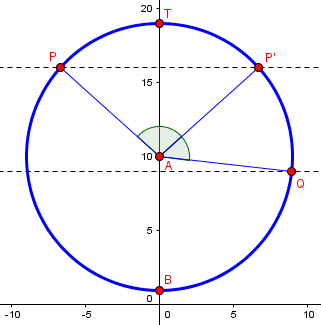Here we have three equal arcs, $$\overset{\frown}{PT}$$, $$\overset{\frown}{TP’}$$, and $$\overset{\frown}{P’Q}$$. That is, $$\angle TAQ$$ is twice $$\angle TAP$$.

That was Sunday night, my time. On Tuesday, Kaushal wrote back:

Thank you so much. I had to submit the answer on Sunday. This is what I did in the end!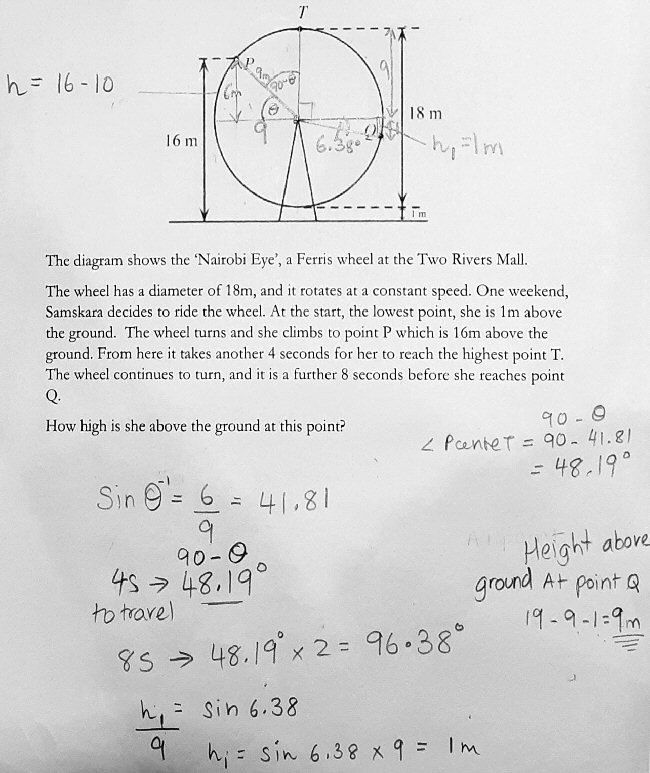He didn’t use the double-angle approach I hinted at, but did use angles rather than the equation of motion, just as I had originally suggested. He has defined angle $$\theta$$ as the complement of $$\angle TAP$$, found its (approximate) value, $$\sin(\theta)=\frac{2}{3}\Rightarrow \theta=\sin^{-1}\left(\frac{2}{3}\right)\approx 41.81$$, and found its complement (I would have just found $$\theta=\cos^{-1}\frac{6}{9} = 48.19^\circ$$). Then he doubled that to get the angle traveled in the next 8 seconds, subtracted 90° to get the angle below the horizontal, and used the sine to answer the question. (I might have used $$9\cos(2\cdot 48.19^\circ) = -1$$.) As I did, he found that the angle happened to be (exactly?) 1 meter below the center.

### Using the double-angle identity

I replied:

Good work. That’s essentially what I initially had in mind when I suggested working directly with angles.

Here’s the extra idea I found: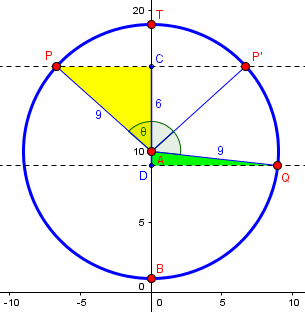Taking θ = ∠PAT, so that ∠TAQ = 2θ. Then

cos θ = 6/9 = 2/3

cos(∠TAQ) = cos 2θ = 2 cos2θ – 1 = 2(4/9) – 1 = -1/9

So AD = AQ cos(∠TAQ) = 9 * -1/9 = -1 (as a signed number).

You don’t need to do anything other than what you did, but this nicely shows that it isn’t a coincidence the answer is so nice; and it makes me suspect they designed the problem this way.

Nothing about this is better than his work, and it is hardly easier; but it is perhaps more fun.

He replied,

Thanks for the extra idea, I hadn’t thought of that.

It’s always fun to find more than one way to solve a problem.

This site uses Akismet to reduce spam. Learn how your comment data is processed.